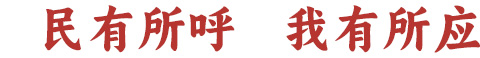问题标题： * 字数在4~20字以内 问题内容： * 请清楚、详细描述您的问题。回车换行 问题类型： 投诉 咨询 建议 反馈   * 请选择正确的问题类型，以方便问题解答。 是否公开： 公开 隐藏   * 查看保密留言。 查询密码： * 此密码可用于查看保密信息及对职能部门回复做出满意度评价,不设密码将不能评价回复。 记者采访： 接受记者采访   * 接受烟台日报传媒集团记者采访会使您的问题得到更快解决。 选择部门： ==请选择== [B]滨州医学院 [B]北海救助局 [B]渤海轮渡公司 [C]财政局 [C]车辆管理所 [C]残联 [C]城管局 [C]城市管理行政执法支队 [C]长岛综合试验区人力资源和社会保障局 [C]长岛综合试验区自然资源局 [C]长岛综合试验区交通和住房建设管理局 [C]长岛综合试验区教育和卫生健康局 [C]长岛综合试验区市场监督管理局 [C]长岛综合试验区综合行政执法局 [D]东昌供热公司 [D]大数据局 [D]档案馆 [D]地方金融监管局 [D]电信公司 [D]动物卫生监督所 [F]福山区不动产登记中心 [F]福山区医保局 [F]福山区交通运输局 [F]福山区农业农村局 [F]福山区水利局 [F]发展改革委 [F]法律援助中心 [F]福山区教体局 [F]福山区民政局 [F]福山区人力资源社会保障局 [F]福山区市场监管局 [F]福山区卫生健康局 [F]福山区文化和旅游局 [F]福山区行政审批服务局 [F]福山区住房建设局 [F]福山区自然资源局 [F]福山区综合行政执法局 [F]妇联 [G]高新区规划国土建设部 [G]高新区卫生健康管理办公室 [G]高新区经济发展部 [G]高新区党群工作部 [G]高新区行政审批服务局 [G]高新区综合行政执法局 [G]工商银行 [G]工业和信息化局 [G]公安局 [G]公安局交警支队 [G]公共就业和人才服务中心 [G]公共资源交易中心 [G]公交集团 [G]公路事业发展中心 [G]公务员局 [G]供电公司 [G]光大银行 [G]国资委 [H]海事局 [H]海洋发展和渔业局 [H]恒丰银行 [H]红十字会 [H]华夏银行 [H]环境监察支队 [H]环境卫生管理中心 [H]海阳市教育和体育局 [H]海阳市公安局 [H]海阳市民政局 [H]海阳市人力资源和社会保障局 [H]海阳市自然资源和规划局 [H]海阳市住房和城乡建设管理局 [H]海阳市交通运输局 [H]海阳市水利局 [H]海阳市农业农村局 [H]海阳市海洋发展和渔业局 [H]海阳市文化和旅游局 [H]海阳市卫生健康局 [H]海阳市行政审批服务局 [H]海阳市市场监督管理局 [H]海阳市综合行政执法局 [H]海阳市医疗保障局 [J]济南铁路局烟台车务段 [J]疾控中心 [J]检察院 [J]建设银行 [J]交通银行 [J]交通运输服务中心 [J]交通运输监察支队 [J]交通运输局 [J]教育局 [K]开发区卫生健康管理办公室 [K]开发区建设交通局 [K]市教育局开发区分局 [K]开发区人力资源社会保障局 [K]开发区市场监管局 [K]开发区行政审批服务局 [K]开发区综合行政执法局 [K]科技局 [K]口岸办 [K]口腔医院 [L]莱山区不动产登记中心 [L]莱山区农业农村局 [L]莱山区医保局 [L]鲁东大学 [L]莱州市教育和体育局 [L]莱州市公安局 [L]莱州市民政局 [L]莱州市人力资源和社会保障局 [L]莱州市自然资源和规划局 [L]莱州市住房和城乡建设管理局 [L]莱州市交通运输局 [L]莱州市水务局 [L]莱州市农业农村局 [L]莱州市海洋发展和渔业局 [L]莱州市文化和旅游局 [L]莱州市卫生健康局 [L]莱州市行政审批服务局 [L]莱州市市场监督管理局 [L]莱州市综合行政执法局 [L]莱州市医疗保障局 [L]龙口市教育和体育局 [L]龙口市公安局 [L]龙口市民政局 [L]龙口市人力资源和社会保障局 [L]龙口市自然资源和规划局 [L]龙口市住房和城乡建设管理局 [L]龙口市交通运输局 [L]龙口市水务局 [L]龙口市农业农村局 [L]龙口市海洋发展和渔业局 [L]龙口市文化和旅游局 [L]龙口市卫生健康局 [L]龙口市行政审批服务局 [L]龙口市市场监督管理局 [L]龙口市综合行政执法局 [L]龙口市医疗保障局 [L]莱阳市教育和体育局 [L]莱阳市公安局 [L]莱阳市民政局 [L]莱阳市人力资源和社会保障局 [L]莱阳市自然资源和规划局 [L]莱阳市住房和城乡建设局 [L]莱阳市交通运输局 [L]莱阳市水务局 [L]莱阳市农业农村局 [L]莱阳市文化和旅游局 [L]莱阳市卫生健康局 [L]莱阳市行政审批服务局 [L]莱阳市市场监督管理局 [L]莱阳市综合行政执法局 [L]莱阳市医疗保障局 [L]莱山区综合行政执法局 [L]莱山区教体局 [L]莱山区民政局 [L]莱山区人力资源和社会保障局 [L]莱山区市场监管局 [L]莱山区卫生健康局 [L]莱山区文化和旅游局 [L]莱山区行政审批服务局 [L]莱山区住房建设局 [L]莱山区自然资源局 [L]劳动监察支队 [L]联通公司 [L]鲁东公证处 [L]旅游公共服务中心 [M]牟平区不动产登记中心 [M] 牟平区医保局 [M]牟平区交通运输局 [M]牟平区水务局 [M]牟平区农业农村局 [M]民政局 [M]牟平区教体局 [M]牟平区民政局 [M]牟平区人力资源社会保障局 [M]牟平区市场监管局 [M]牟平区卫生健康局 [M]牟平区文化和旅游局 [M]牟平区行政审批服务局 [M]牟平区住房建设局 [M]牟平区自然资源局 [M]牟平区综合行政执法局 [N]农业农村局 [N]农业银行 [P]蓬莱区不动产登记中心 [P]蓬莱区交通运输局 [P]蓬莱区教育和体育局 [P]蓬莱区民政局 [P]蓬莱区农业农村局 [P]蓬莱区人社局 [P]蓬莱区市场监管局 [P]蓬莱区水务局 [P]蓬莱区卫生健康局 [P]蓬莱区文化和旅游局 [P]蓬莱区行政审批服务局 [P]蓬莱区医保局 [P]蓬莱区住房和城乡建设管理局 [P]蓬莱区自然资源和规划局 [P]蓬莱区综合行政执法局 [P]排水服务中心 [P]平安财险公司 [Q]清泉供热 [Q]栖霞市教育和体育局 [Q]栖霞市公安局 [Q]栖霞市民政局 [Q]栖霞市人力资源和社会保障局 [Q]栖霞市自然资源和规划局 [Q]栖霞市住房和城乡建设局 [Q]栖霞市交通运输局 [Q]栖霞市水务局 [Q]栖霞市农业农村局 [Q]栖霞市文化和旅游局 [Q]栖霞市卫生健康局 [Q]栖霞市行政审批服务局 [Q]栖霞市市场监督管理局 [Q]栖霞市综合行政执法局 [Q]栖霞市医疗保障局 [Q]奇山医院 [Q]气象局 [R]热力公司 [R]人保财险公司 [R]人民防空办公室 [R]人力资源社会保障局 [R]人民银行烟台市中心支行 [S]山东高速烟台发展公司 [S]市中心血站 [S]市农业技术推广中心 [S]市生态环境局蓬莱分局 [S]市不动产登记中心 [S]市住房保障和房产交易中心 [S]市安全生产监察支队 [S]山东工商学院 [S]市教育局高新区分局 [S]数字化城市管理服务中心（城市公用事业服务中心） [S]三站批发交易市场管理服务中心 [S]山东广电网络有限公司烟台分公司 [S]商务局 [S]社保中心 [S]审计局 [S]生态环境局 [S]省农村信用联社烟台办事处 [S]市场监管局 [S]市场监管局执法支队 [S]市法院 [S]市民卡有限公司 [S]市生态环境局福山分局 [S]市生态环境局开发区分局 [S]市生态环境局莱山分局 [S]市生态环境局牟平分局 [S]市生态环境局芝罘分局 [S]市政养护中心 [S]水利局 [S]税务局 [S]司法局 [T]太平洋财险公司 [T]太平洋人寿公司 [T]体育局 [T]团市委 [T]退役军人局 [W]500供热公司 [W]外办 [W]卫生计生监督所 [W]卫生健康委 [W]文化和旅游局 [W]文化和旅游市场执法支队 [X]消防救援支队 [X]消费者投诉中心 [X]新奥燃气公司 [X]行政审批服务局 [Y]业达热电公司 [Y]烟台大学 [Y]烟草专卖局 [Y]烟台港集团 [Y]烟台国际机场集团 [Y]烟台海关 [Y]烟台农村商业银行 [Y]烟台交运 [Y]烟台山医院 [Y]烟台银行 [Y]医保局 [Y]移动公司 [Y]银保监分局 [Y]应急管理局 [Y]邮政储蓄银行 [Y]邮政公司 [Y]邮政管理局 [Y]毓璜顶医院 [Y]园林建设养护中心 [Z]芝罘区综合行政执法局 [Z]知识产权局 [Z]中国人寿公司 [Z]中国银行 [Z]中信银行 [Z]中医医院 [Z]仲裁办 [Z]住房城乡建设局 [Z]住房公积金管理中心 [Z]自来水公司 [Z]自然资源和规划局 [Z]总工会 [Z]质监局 [Z]芝罘区不动产登记中心 [Z]芝罘区医保局 [Z]芝罘区农业农村局 [Z]芝罘医院 [Z]招远市教育和体育局 [Z]招远市公安局 [Z]招远市民政局 [Z]招远市人力资源和社会保障局 [Z]招远市自然资源和规划局 [Z]招远市住房和城乡建设局 [Z]招远市交通运输局 [Z]招远市水务局 [Z]招远市农业农村局 [Z]招远市文化和旅游局 [Z]招远市卫生健康局 [Z]招远市行政审批服务局 [Z]招远市市场监督管理局 [Z]招远市综合行政执法局 [Z]招远市医疗保障局 [Z]招商银行 [Z]芝罘区教体局 [Z]芝罘区民政局 [Z]芝罘区人力资源社会保障局 [Z]芝罘区市场监管局 [Z]芝罘区卫生健康局 [Z]芝罘区文化和旅游局 [Z]芝罘区行政审批服务局 [Z]芝罘区住房建设局 [Z]芝罘区自然资源局   * 各部门按照部门首个字拼音字母顺序排序。 电话对部门公开： 公开 不公开   * 为方便有关部门调查取证，提高问题解决效率和效果，建议公开。 隐私声明： 如需要记者对您所提供的线索进行采访，请填写您的正确联系方式以方便烟台日报传媒集团记者及时准确能联系到您进行相关采访。对于您的联系方式我们将进行严格保密。 真实姓名： 手机号： * 手机号必填 短信验证码： * 验证码必填 QQ号： 电子邮箱： 图片附件： * 请上传8M以下的图片文件，最多可添加4张。 短视频： 建议上传15秒内MP4(H264)格式短视频，视频体积不大于40M。

•水母网官网微信
•水母网官网微博